#### Thank you for registering.

One of our academic counsellors will contact you within 1 working day.

Please check your email for login details.

Click to Chat

1800-1023-196

+91-120-4616500

CART 0

• 0
MY CART (5)

Use Coupon: CART20 and get 20% off on all online Study Material

ITEM
DETAILS
MRP
DISCOUNT
FINAL PRICE
Total Price: Rs.

There are no items in this cart.
Continue Shopping• Complete JEE Main/Advanced Course and Test Series
• Offered Price: Rs. 15,900
• View Details

# Chapter 4: Triangles Exercise – 4.2

### Question: 1

In a Δ ABC, D and E are points on the sides AB and AC respectively such that DE ∥∥ BC.

1.) If AD = 6 cm, DB = 9 cm and AE = 8 cm, Find AC.

2.) If AD/DB = 3/4 and AC = 15 cm, Find AE.

3.) If AD/DB = 2/3 and AC = 18 cm, Find AE.

4.) If AD = 4 cm, AE = 8 cm, DB = x - 4 cm and EC = 3x – 19, find x.

5.) If AD = 8 cm, AB = 12 cm and AE = 12 cm, find CE.

6.) If AD = 4 cm, DB = 4.5 cm and AE = 8 cm, find AC.

7.) If AD = 2 cm, AB = 6 cm and AC = 9 cm, find AE.

8.) If AD/BD = 4/5 and EC = 2.5 cm, Find AE.

9.) If AD = x cm, DB = x – 2 cm, AE = x + 2 cm, and EC = x -1 cm, find the value of x.

10.) If AD = 8x - 7 cm, DB = 5x - 3 cm, AE = 4x - 3 cm, and EC = (3x – 1) cm, Find the value of x.

11.) If AD = 4x – 3, AE = 8x – 7, BD = 3x - 1, and CE = 5x – 3, find the value of x.

12.) If AD = 2.5 cm, BD = 3.0 cm, and AE = 3.75 cm, find the length of AC.

### Solution:

1) It is given that Δ ABC AND DE ∥ BC

We have to find AC,

Since, AD = 6 cm,

DB = 9 cm and AE = 15 cm.

AB = 15 cm.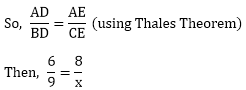6x = 72 cm

x = 72/6 cm

x = 12 cm

Hence, AC = 12 + 8 = 20.

2. It is given that AD/BD = 3/4 and AC = 15 cm

We have to find out AE,

Let, AE = x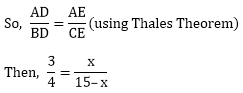45 – 3x = 4x

- 3x – 4x = – 45

7x = 45

x = 45/7

x = 6.43 cm

3. It is given that AD/BD = 2/3 and AC = 18 cm

We have to find out AE,

Let, AE = x and CE = 18 – x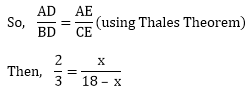3x = 36 – 2x

5x = 36 cm

X = 36/5 cm

X = 7.2 cm

Hence, AE = 7.2 cm

4. It is given that AD = 4 cm, AE = 8 cm, DB = x – 4 and EC = 3x  – 19

We have to find x,4(3x – 19) = 8(x – 4)

12x – 76 = 8(x – 4)

12x – 8x = – 32 + 76

4x = 44 cm

X = 11 cm

5. It is given that AD = 8 cm, AB = 12 cm, and AE = 12 cm.

We have to find CE,8CE = 4 × 12 cm

CE = (4 × 12)/8 cm

CE = 48/8 cm

CE = 6 cm

6. It is given that AD = 4 cm, DB = 4.5 cm, AE = 8 cm

We have to find out ACAC = 9 cm

7. It is given that AD = 2 cm, AB = 6 cm, and AC = 9 cm

We have to find out AE

DB = 6 – 2 = 4 cm4x = 18 – 2x

6x = 18

x = 3 cm

8. It is given that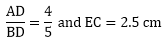We have to find out AE9. It is given that AD = x, DB = x – 2, AE = x + 2 and EC = x – 1

We have to find the value of x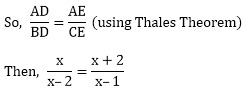X(x – 1) = (x – 2)(x + 2)

x2 – x – x2 + 4 = 0

x = 4

10. It is given that AD = 8x - 7, DB = 5x – 3, AER = 4x – 3 and EC = 3x -1

We have to find the value of x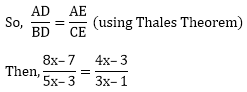(8x – 7)(3x – 1) = (5x – 3)(4x – 3)

24x2 – 29x + 7 = 20x– 27x + 9

4x2 – 2x – 2 = 0

2(2x2 – x – 1) = 0

2x2 – x – 1 = 0

2x2 – 2x + x – 1 = 0

2x(x – 1) + 1(x – 1) = 0

(x – 1)(2x + 1) = 0

X = 1 or x = -1/2

Since the side of triangle can never be negative

Therefore, x = 1.

11. It is given that AD = 4x – 3, BD = 3x – 1, AE = 8x – 7 and EC = 5x – 3

For finding the value of x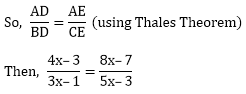(4x – 3)(5x – 3) = (3x – 1)(8x – 7)

4x(5x – 3) -3(5x – 3) = 3x(8x – 7) -1(8x – 7)

20x2 – 12x – 15x + 9 = 24x2 – 29x + 7

20x2 -27x + 9 = 242 -29x + 7

Then,

- 4x2 + 2x + 2 = 0

4x2 – 2x – 2 = 0

4x2 – 4x + 2x – 2 = 0

4x(x – 1) + 2(x – 1) = 0

(4x + 2)(x – 1) = 0

x = 1 or x = - 2/4

Since, side of triangle can never be negative

Therefore x = 1

12. It is given that, AD = 2.5 cm, AE = 3.75 cm and BD = 3 cm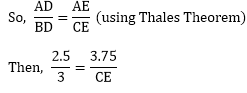2.5CE = 3.75 × 3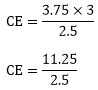CE = 4.5

Now, AC = 3.75 + 4.5

AC = 8.25 cm.

### Question: 2

In a Δ ABC, D and E are points on the sides AB and AC respectively. For each of the following cases show that DE ∥ BC.

1.) AB = 12 cm, AD = 8 cm, AE = 12 cm, and AC = 18 cm.

2.) AB = 5.6 cm, AD = 1.4 cm, AC = 7.2 cm, and AE = 1.8 cm.

3.) AB = 10.8 cm, BD = 4.5 cm, AC = 4.8 cm, and AE = 2.8 cm.

4.) AD = 5.7 cm, BD = 9.5 cm, AE = 3.3 cm, and EC = 5.5 cm.

### Solution:

1)  It is given that D and R are the points on sides AB and AC.

We have to find that DE ∥ BC.

Acc. To Thales Theorem,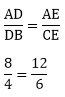2 = 2 (LHS = RHS)

Hence, DE ∥ BC.

2)  It is given that D and E are the points on sides AB and AC

We need to prove that DE ∥ BC

Acc. To Thales Theorem,Hence, DE ∥ BC.

3)  It is given that D and E are the points on sides AB and AC.

We need to prove DE ∥ BC.

Acc. To Thales Theorem,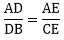AD = AB – DB = 10.8 – 4.5 = 6.3

And,

EC = AC – AE = 4.8 – 2.8 = 2

Now,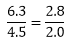Hence, DE ∥ BC.

4) It is given that D and E are the points on sides AB and Ac.

We need to prove that DE ∥∥ BC.

Acc. To Thales Theorem,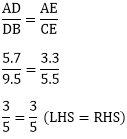Hence, DE ∥ BC.

### Question: 3

In a Δ ABC, P and Q are the points on sides AB and AC respectively, such that PQ ∥ BC. If AP = 2.4 cm, AQ = 2 cm, QC = 3 cm, and BC = 6 cm, Find AB and PQ.

### Solution:

It is given that AP = 2.4 cm, AQ = 2 cm, QC = 3 cm, and BC = 6 cm.

We need to find AB and PQ.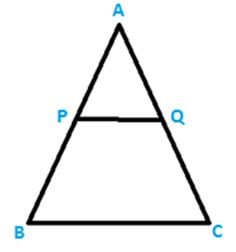Using Thales Theorem,2PB = 2.4 × 3 cm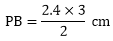PB = 3.6 cm

Now, AB = AP + PB

AB = 2.4 + 3.6

AB = 6 cm

Since, PQ ∥ BC, AB is transversal, then,

Δ APQ = Δ ABC    (by corresponding angles)

Since, PQ ∥ BC, AC is transversal, then,

Δ APQ = Δ ABC    (by corresponding angles)

In Δ ABQ and Δ ABC,

∠APQ = ∠ABC

∠AQP = ∠ACB

Therefore, Δ APQ = Δ ABC (angle similarity)

Since, the corresponding sides of similar triangles are proportional,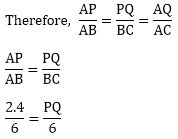Therefore, PQ = 2.4 cm.

### Question: 4

In a Δ ABC, D and E are points on AB and AC respectively, such that DE ∥ BC. If AD = 2.4 cm, AE = 3.2 cm, DE = 2 cm, and BC = 5 cm. Find BD and CE.

### Solution:

It is given that AD = 2.4 cm, AE = 3.2 cm, DE = 2 cm and BE = 5 cm.

We need to find BD and CE.

Since, DE ∥ BC, AB is transversal, then,

∠APQ = ∠ABC

Since, DE ∥ BC, AC is transversal, then,

∠AED = ∠ACB

In Δ ADE and Δ ABC,

∠ADE = ∠ABC

∠AED = ∠ACB

So, Δ ADE = Δ ABC (angle angle similarity)

Since, the corresponding sides of similar triangles are proportional, then,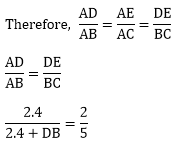2.4 + DB = 6

DB = 6 – 2.4

DB = 3.6 cm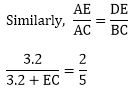3.2 + EC = 8

EC = 8 – 3.2

EC = 4.8 cm

Therefore, BD = 3.6 cm and CE = 4.8 cm.

### Question: 5

In figure given below, state PQ ∥ EF.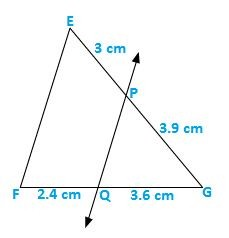### Solution:It is given that EP = 3 cm, PG = 3.9 cm, FQ = 3.6 cm and QG = 2.4 cm

We have to check that PQ ∥ EF or not.

Acc. to Thales Theorem,As we can see it is not prortional.

So, PQ is not parallel to EF.

### Question: 6

M and N are the points on the sides PQ and PR respectively, of a ΔPQR. For each of the following cases, state whether MN ∥ QR.

(i)  PM = 4 cm, QM = 4.5 cm, PN = 4 cm, NR = 4.5 cm.

(ii) PQ = 1.28 cm, PR = 2.56 cm, PM = 0.16 cm, PN = 0.32 cm.

### Solution:

(i) It is given that PM = 4 cm, QM = 4.5 cm, PN = 4 cm, and NR = 4.5 cm.

We have to check that MN ∥ QR or not.

Acc. to Thales Theorem,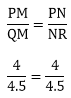Hence, MN ∥ QR.

(ii) It is given that PQ = 1.28 cm, PR = 2.56 cm, PM = 0.16 cm, and PN = 0.32 cm.

We have to check that MN ∥ QR or not.

Acc. to Thales Theorem,Hence, MN ∥ QR.

### Question: 7

In three line segments OA, OB, and OC, points L, M, N respectively are so chosen that LM ∥ AB and MN ∥ BC but neither of L, M, and N nor A, B, C are collinear. Show that LN ∥ AC.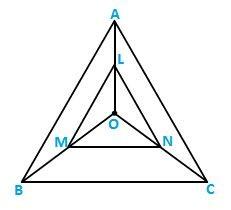### Solution:In ΔOAB, Since, LM ∥ AB,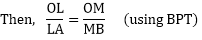In Δ OBC, Since, MN ∥ BC,From the above equations,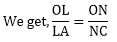In a Δ OCA,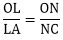LN ∥ AC (by converse BPT)

### Question: 8

If D and E are the points on sides AB and AC respectively of a ΔABC such that DE ∥ BC and BD = CE. Prove that Δ ABC is isosceles.

### Solution:

It is given that in Δ ABC, DE ∥ BC and BD = CE.

We need to prove that Δ ABC is isosceles.

Acc. to Thales Theorem,AD = AE

Now, BD = CE and AD = AE.

So, AD + BD = AE + CE.

Therefore, AB = AC.

Therefore, Δ ABC is isosceles.## Complete JEE Main/Advanced Course and Test Series

OFFERED PRICE: Rs. 15,900
View Details Xpress Buy

### Course Features

• 731 Video Lectures
• Revision Notes
• Test paper with Video Solution
• Mind Map
• Study Planner
• NCERT Solutions
• Discussion Forum
• Previous Year Papers

### Course Features

• 728 Video Lectures
• Revision Notes
• Previous Year Papers
• Mind Map
• Study Planner
• NCERT Solutions
• Discussion Forum
• Test paper with Video Solution